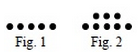### Home > MC1 > Chapter 10 > Lesson 10.2.3 > Problem10-102

10-102.

Draw three figures of a pattern of dots that can be represented by the rule $y = 3x + 2$, where $x$ represents the figure number and $y$ represents the number of dots.

The first two figures of a pattern have been drawn for you.